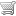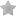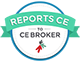No items in your cart.

# Linear Regression Analysis (Online CE Course)(based on 223 customer ratings)

How to Subscribe
 MLS & MLT Comprehensive CE PackageIncludes 126 CE courses, most popular \$95Add to cart Pick Your CoursesUp to 8 CE hours \$50Add to cart Individual course \$20Add to cart

The purpose of this course is to demonstrate how to use linear regression to predict the value of one variable, given the value of the other variable and the experimental data concerning the relationship between the variables.

See all available courses »

### Continuing Education CreditsP.A.C.E.® Contact Hours (acceptable for AMT, ASCP, and state recertification): 2.5 hour(s)Course number 578-057-18, approved through 9/30/2020Florida Board of Clinical Laboratory Personnel Credit Hours - Supervision/Administration, Quality Control/Quality Assurance, and Safety: 2.5 hour(s)Course number 20-676420, approved through 9/1/2020

### Objectives

• Define linear regression and explain how it is used.
• Given data points that fall on a straight line, find the equation for the line.
• Use the regression equation to predict the value of a dependent variable give the value of the independent variable.
• Explain what is meant by the phrase "line of best fit."
• Given a set of data, determine the best fit using the least squares method.
• Define and calculate standard error of estimate.
• Explain the difference between a, alpha, b, and beta, as applied to regression analysis, and describe why confidence intervals are calculated for the slope and y-intercept.

### Customer Ratings(based on 223 customer ratings)

### Course Outline

Click on the links below to preview selected pages from this course.
• Introduction to Regression Analysis
• Predicting a Value
• A Regression Analysis Example
• A Regression Analysis Example, continued
• Calculating the Y-Intercept
• Prediction Using the Resulting Equation
• Given the following creatinine standards:mg/dLAbsorbance30.1460.2690.38What is the correct form of the regression line?
• Given the data and linear regression line you calculated on the previous question, what is the expected absorbance of a 10 mg/dL sample?
• True or false: you should make a scatterplot of your data before you calculate the regression line.
• A scatterplot of the data below is shown on the right, confirming a linear relationship. Given the following data, calculate the regression line.xy2 9...
• Introduction to Least Squares Method of Best Fit
• Introduction to Least Squares Method
• The Least Squares Line
• Standard Error of Estimate
• Calculate the sum of squares for line B. To do this, you must calculate , the difference y- , and the squared difference (y-)2 for each point, and the...
• Using the sum of squares from the previous question (440.25), calculate the Standard Error of Estimate for line B to the nearest thousandth using the ...
• Least Squares Calculation
• Determining the Least Squares Line
• Formulae for Determining the Slope and Intercept
• Calculating the Standard Error of Estimate
• Correlation Coefficient
• Example Regression Line Calculation
• Using the Least Squares Formulae
• Determining Se and r2
• Data for Questions
• Using the data, calculate the total of the (x-)(y-) values. What is the total (rounded to the nearest whole number)?PointReference Method (x)Test Meth...
• Using the same data, calculate the total of the (x-)2 values. What is the total?The average of x is 20 and the average of y is 23.8.PointReference Met...
• Using the formulas below and the information from the previous questions (shown again in the table below), what are the slope and y-intercept of the l...
• What is the Standard Error of Estimate for this regression line, using the shortcut form of the equation shown below:a = 2.4b = 1.070= 23.8PointRefere...
• Calculation of Confidence Intervals for Least Squares
• References
• References

Level of instruction: Intermediate

Intended audience: This course is appropriate for laboratory professionals, and for students in clinical laboratory science programs who want a review of the statistics that are analyzed for assessment of quality control.

Author Information:  Mary Ann Fiene, MT(ASCP), has authored several articles on the subjects of curriculum development, competency evaluation, and job restructuring. Her articles have appeared in the Journal of Allied Health, American Journal of Medical Technology (now published as Clinical Laboratory Science), and Medical Laboratory Observer.

Ms. Fiene was affiliated as an educator with the Kettering Medical Center School of Medical Technology.

Alan Reichert, PhD, is a professor of finance at Cleveland State University in Ohio.

About the Course: This course is part of a series of courses adapted for the web by MediaLab Inc. under license from Educational Materials for Health Professionals Inc. Dayton OH, 45420. Copyright EMHP. The course was reviewed and revised in 2012.

### Keywords

These are the most common topics and keywords covered in Linear Regression Analysis:

How to Subscribe
 MLS & MLT Comprehensive CE PackageIncludes 126 CE courses, most popular \$95Add to cart Pick Your CoursesUp to 8 CE hours \$50Add to cart Individual course \$20Add to cart